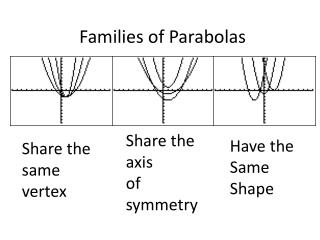DownloadDownload PresentationFamilies of Parabolas

# Families of Parabolas

Télécharger la présentation## Families of Parabolas

- - - - - - - - - - - - - - - - - - - - - - - - - - - E N D - - - - - - - - - - - - - - - - - - - - - - - - - - -
##### Presentation Transcript

1. Families of Parabolas Share the axis of symmetry Have the Same Shape Share the same vertex

2. Graph each group of equations on the same screen.Compare and contrast the graphs.What conclusions can be drawn? y = x2 y =0.2x2 y =3x2

3. Your graph should look like this y = 3x2 y = x2 y = 0.2x2

4. Graph each group of equations on the same screen.Compare and contrast the graphs.What conclusions can be drawn? y = x2 y =x2-6 y =x2+3

5. Your graph should look like this y=x2+3 y = x2 y = x2-6

6. Graph each group of equations on the same screen.Compare and contrast the graphs.What conclusions can be drawn? y = x2 y =(x + 2)2 y =(x -4)2

7. Your graph should look like this y=(x-4)2 y = (x+2)2 y=x2

8. Graph each group of equations on the same screen.Compare and contrast the graphs.What conclusions can be drawn? y = x2 y =(x-7)2+2

9. Your graph should look like this

10. Your Turn Graph each group of equations on the same screen.Compare and contrast the graphs.What conclusions can be drawn? y = x2 y =2x2 y= 4x2

11. Your Turn Graph each group of equations on the same screen.Compare and contrast the graphs.What conclusions can be drawn? y = x2 y =x2 -1 y= x2 -8

12. Your Turn Graph each group of equations on the same screen.Compare and contrast the graphs.What conclusions can be drawn? y = x2 y =-x2

13. Graph each group of equations on the same screen.Compare and contrast the graphs.What conclusions can be drawn? y = -x2 y =-(x+2)2 y =-(x+4)2

14. General Rules For Shifts in Parabolas The parent parabola is y = x2 • For y = a x2 • if a is positive the parabola will open upward • if a is negative the parabola will open downward • as a increase in absolute value the parabola gets more narrow 2. For y = (x+b)2 shifts the graph to the left b units For y = (x-b)2 shifts the graph to the right b units

15. General Rules for 3. For y = x2 +cshifts the graph upward b units For y = x2 -c shifts the graph downward b units 4.You can have a combination of shifts y = (x + b)2 –c will shift the graph of y = x2b units to the left and c units downward

16. One Last Problem In a computer game, a player dodges spaced shuttles that are shaped like parabolas. Suppose the vertex of one shuttle is at the origin. The shuttle’s initial shape and position are given by the equation y = 0.5x2. It leaves the screen with its vertex at (6,5). Find an equation to model the final shape of the shuttle.

17. HOMEWORK Page 466 #14 – 34 EVENS